Question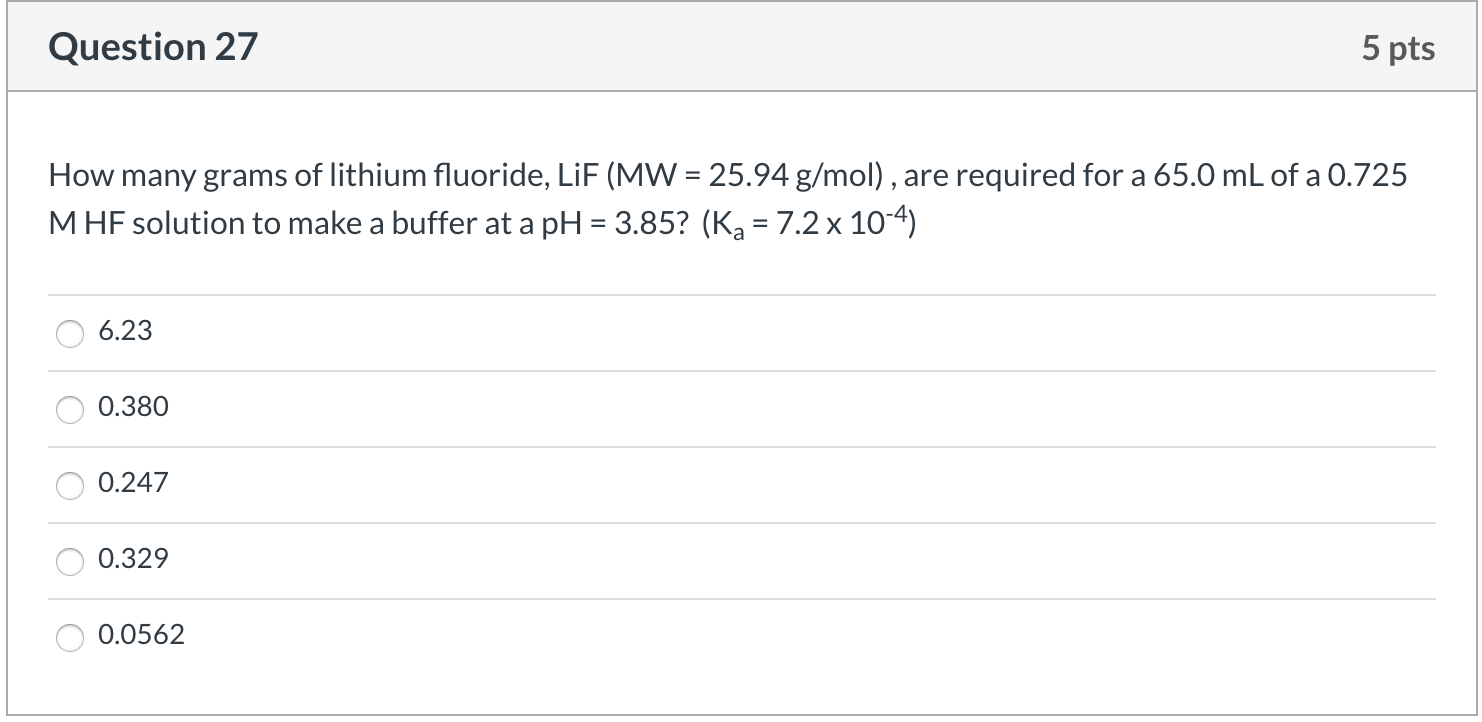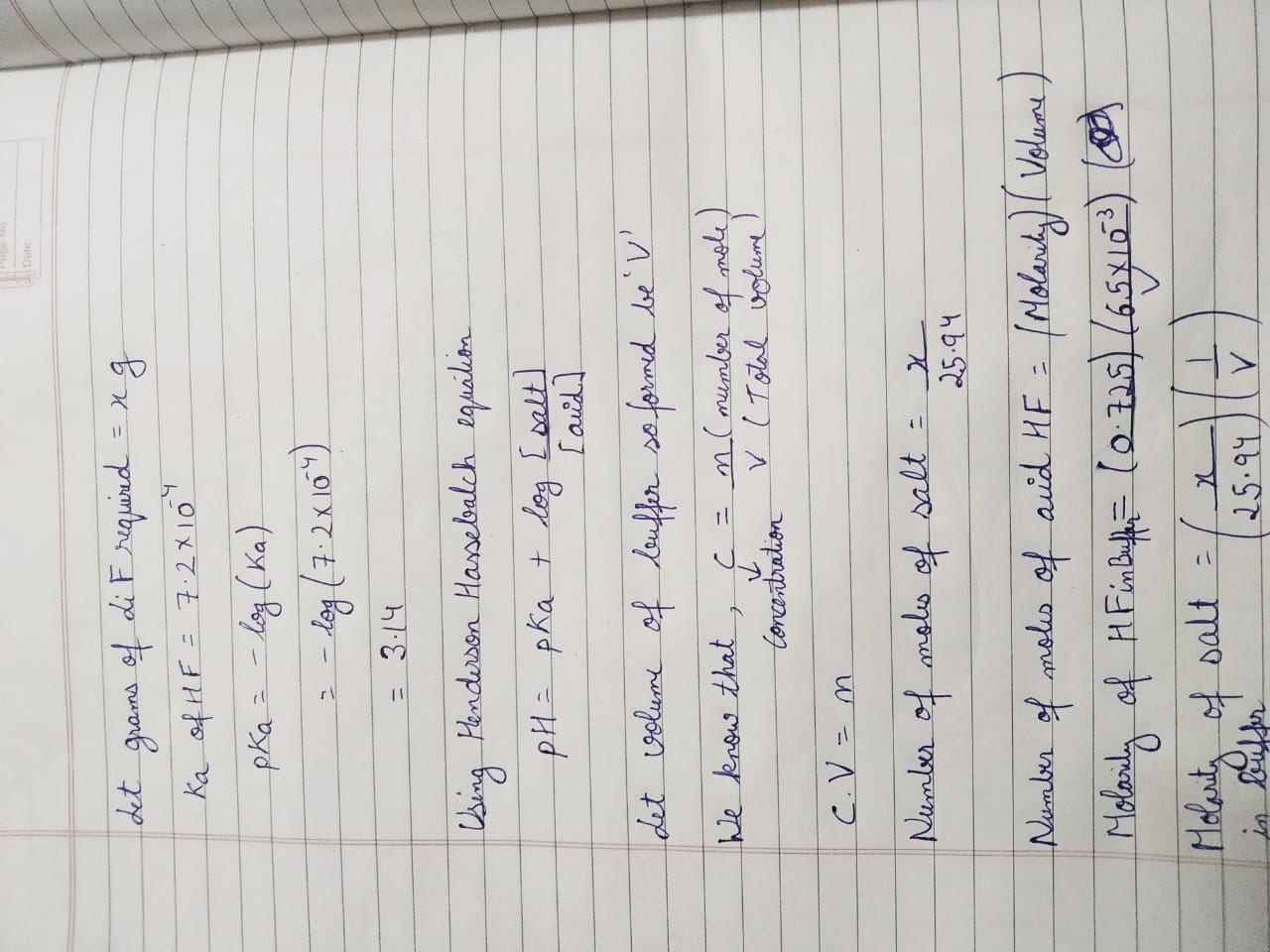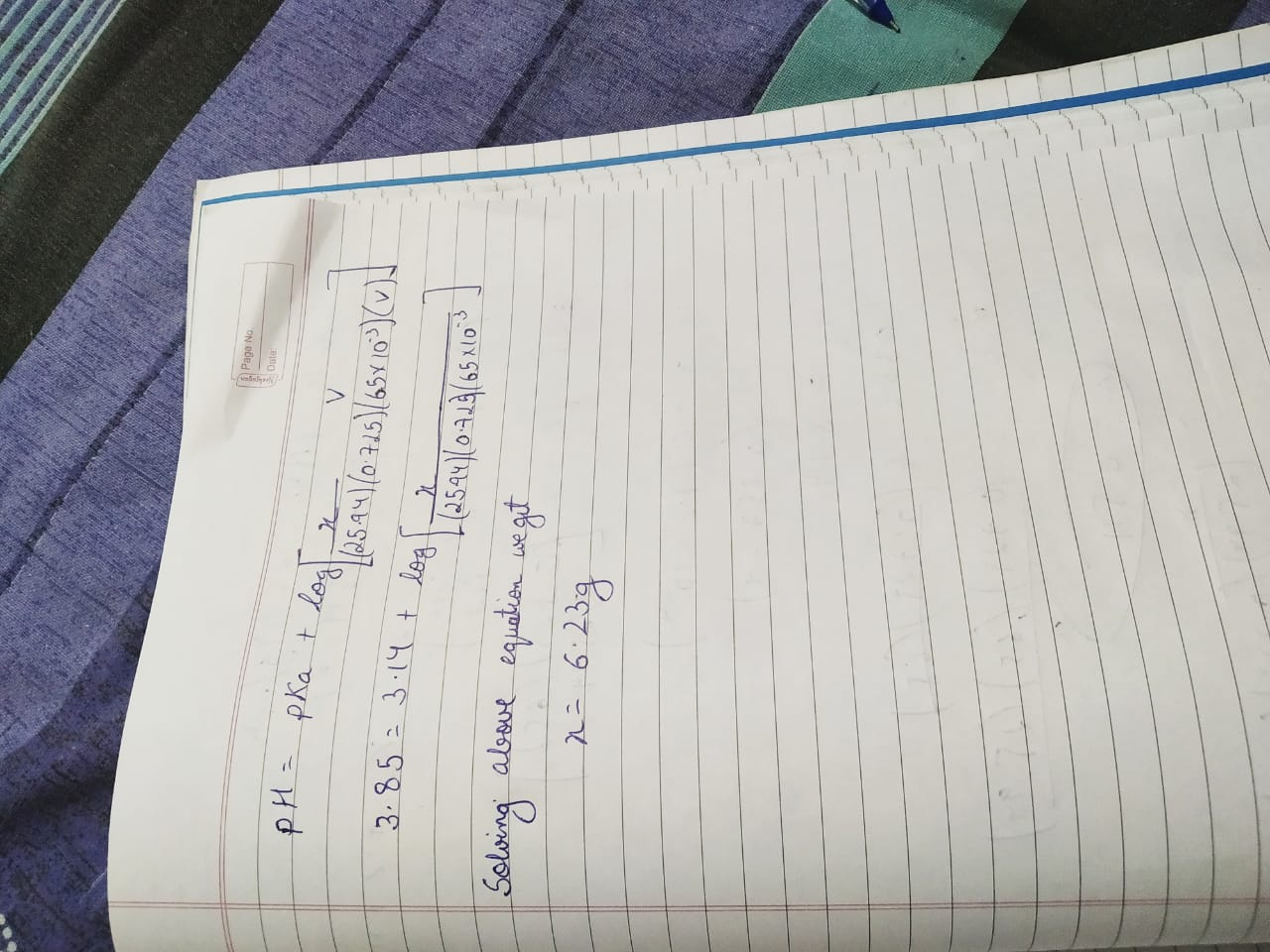#### Earn Coins

Coins can be redeemed for fabulous gifts.

Similar Homework Help Questions
• ### How many grams of dipotassium succinate trihydrate (K2C4H4O4·3H2O, MW = 248.32 g/mol) must be added to...

How many grams of dipotassium succinate trihydrate (K2C4H4O4·3H2O, MW = 248.32 g/mol) must be added to 650.0 mL of a 0.0420 M succinic acid solution to produce a pH of 5.867? Succinic acid has pKa values of 4.207 (pKa1) and 5.636 (pKa2).

• ### QUESTION 5                  8 MARKS a) How many grams of BaCl2.2H2O (MW = 244.27 g/mol) you need...

QUESTION 5                  8 MARKS a) How many grams of BaCl2.2H2O (MW = 244.27 g/mol) you need to prepare 250 mL of 0.15 M chloride ion solution? 2 marks b) It was established that this reagent was contaminated by CaCl2 (MW = 181.82 g). The content of calcium in the solid sample was found to be 4750 ppm (w/w). What was the percentage decrease in the molar Barium ion concentration caused by this contamination? 6 marks hi chemists I recieved this...

• ### (3 pts) 4. You are asked to prepare a 0.100 M)glycine buffer pH 9.0. You are given a bottle of glycine (FW 75.1 g/mol) and a stock solution of 1.00 M NaOH. How many grams of glycine and how many...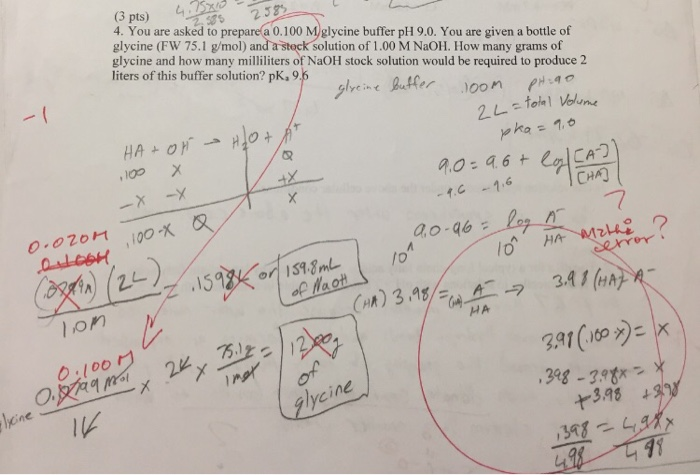(3 pts) 4. You are asked to prepare a 0.100 M)glycine buffer pH 9.0. You are given a bottle of glycine (FW 75.1 g/mol) and a stock solution of 1.00 M NaOH. How many grams of glycine and how many milliliters of NaOH stock solution would be required to produce 2 liters of this buffer solution? pk,95 alyeine tr oon 2 38 CHA) 7 3A1 (HA o.l0o glycine 3.98 (3 pts) 4. You are asked to prepare a 0.100 M)glycine...

• ### D Question 4 5 pts 2b) There are 0.10 moles of N2O4 and 0.20 moles of...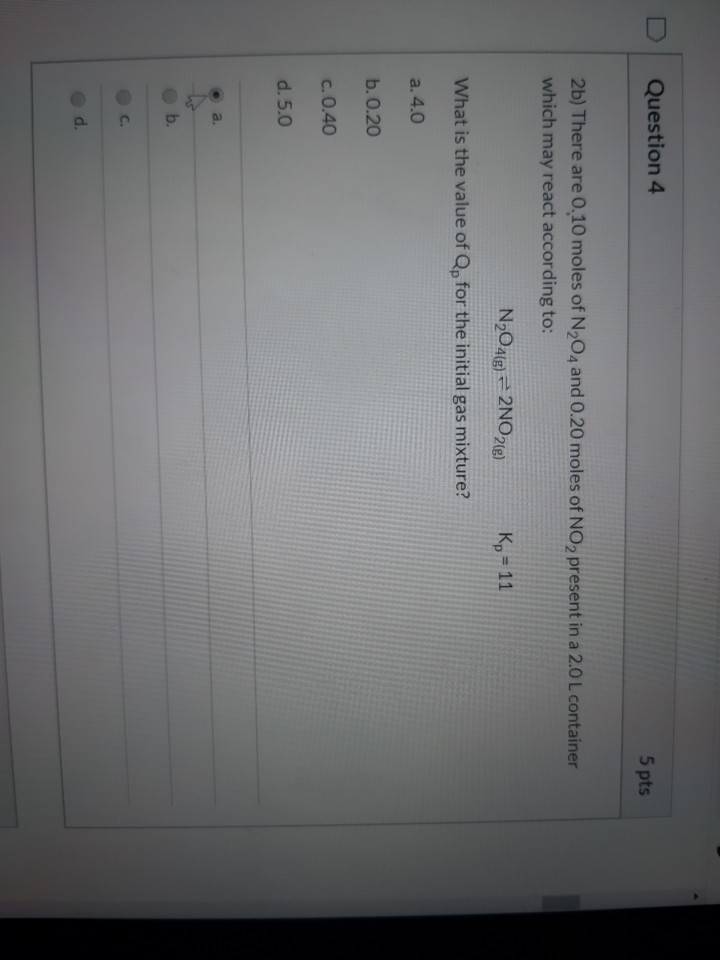D Question 4 5 pts 2b) There are 0.10 moles of N2O4 and 0.20 moles of NO 2 present in a 2.0 L container which may react according to: N2O4(g) = 2N028) Kp = 11 What is the value of Qp for the initial gas mixture? a. 4.0 b. 0.20 c. 0.40 d.5.0 . a. b. Consider the following equilibrium systems. Which direction will the reactions shift (reactants or products) when cooled? 200g + O2(g) = 2CO2(g) H2e+12(e)=2HI® H2(g) +126)...